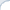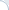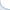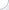English»Software»Machine Learning»Bayesian and deep Bayesian | searchivarius.orgBMR: Bayesian Multinomial Regression Software Edward   - A library for probabilistic modeling, inference, and criticism. Deep generative models, variational inference. Runs on TensorFlow. Flexible Bayesian Modelling.   - This software supports Bayesian regression and classification models based on neural networks and Gaussian processes, and Bayesian density estimation and clustering using mixture models and Dirichlet diffusion trees. It also supports a variety of Markov chain sampling methods, which may be applied to distributions specified by simple formulas, including simple Bayesian models defined by formulas for the prior and likelihood. GPyTorch Method home for gp-mc-1   - Gaussian processes for regression trained using a fully Bayesian approach using an MCMC implementation. probtorch   - Probabilistic Torch is library for deep generative models that extends PyTorch. PyMC & PyMC3   - python modules that implement Bayesian statistical models and fitting algorithms, including Markov chain Monte Carlo. Pyro   - Deep universal probabilistic programming with Python and PyTorch Spearmint   - a package to perform Bayesian optimization. The Libra toolkit   - a collection of algorithms for learning and inference with discrete probabilistic models, including Bayesian networks (BNs), Markov networks (MNs), dependency networks (DNs), sum-product networks (SPNs), and arithmetic circuits (ACs). Compared to other toolkits, Libra focuses more on structure learning, especially for tractable models in which exact inference is efficient.